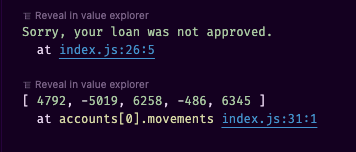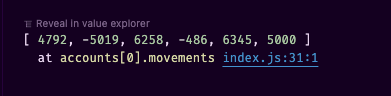# const - JS | lectureWorking With Arrays

some and every

In this lecture, we will learn about the `some` and `every` methods. And to start learning about the `some` method, let's look back at the `includes` method we studied earlier.

Let's consider once more our `movements` array and execute the below code:

script.js
``````const movements = [200, 450, -400, 3000, -650, -130, 70, 1300];

console.log(movements.includes(-130)); // true``````

We get true because the `includes` method checks if an array contains a certain value. Now, however, is use to test only for equality. So basically, the includes method above returns `true` if any value in the array is exactly equal to `-130`. But what if we wanted to test for a condition? That's where the `some` method comes in.

Let's say we would like to know if there is any positive movement in the array. i.e., any number above zero. To do that we will use the some method and pass in a callback function. The callback function will receive the current element as an argument. And we will return a boolean value. So if the current element is greater than zero, we will return true. Otherwise, we will return false.

script.js
``````console.log(movements.some((mov) => mov > 0)); // true

/* Other examples */

// Check if there is any movement greater than 5000
console.log(movements.some((mov) => mov > 5000)); // false

// Check if there is any movement greater than 1500
console.log(movements.some((mov) => mov > 1500)); // true``````

You can see that both the `some` and `includes` methods looks kind of similar. But as mentionned above, the difference is that `includes` checks for equality but with `some`, we can test for any condition. So we could rewrite the `includes` method above using the `some` method like this:

script.js
``console.log(movements.some((mov) => mov === -130)); // true``

If you really just need to test for equality, it doesn' make much sense to use the `some` method. You can simply use the `includes` method. But if you need to test for a condition (`mov > 5000`), like in the examples above, then the `some` method is the way to go.

Let's have a more concrete example. Let's consider our bank app we introduced in the previous lecture, and let's say we want to implement a loan feature. And a loan is only approved, if there is any deposit that's greater or equal to 10% of the requested loan amount.

Might sound complicated, but it's actually pretty simple. Before implementing the loan feature, let's consider the below user accounts.

script.js
``````const accounts = [
{
owner: 'Floyd Norris',
movements: [4792, -5019, 6258, -486, 6345],
},
{
name: 'Lee Shelton',
movements: [612, 4346, 407, 5439, 350],
},
{
name: 'Donald Ramsey',
movements: [4408, -4640, 2100, 5687, -2383],
},
];``````

Let's now implement the loan feature. In order to make a loan, our function will need to know the account requesting the loan and the amount of the loan.

script.js
``````const requestLoan = function (account, loanAmmount) {  // do something
};``````

Next, we must check if the loan amount is greater than zero and if the account has any deposit that's greater or equal to 10% of the loan amount. Whenever you hear or see the word any, then it's probably a good use case for the `some` method.

script.js
``````const requestLoan = function (account, loanAmmount) {
if (    loanAmmount > 0 &&    account.movements.some((mov) => mov >= loanAmmount * 0.1)  ) {    // do something
}
};``````

Now, all that's left is to add the loan amount to the account's movements.

script.js
``````const requestLoan = function (account, loanAmmount) {
if (
loanAmmount > 0 &&
account.movements.some((mov) => mov >= loanAmmount * 0.1)
) {
account.movements.push(loanAmmount);  }
};``````

Let's also add an else statement so that we can log a message if the loan is not approved.

script.js
``````const requestLoan = function (account, loanAmmount) {
if (
loanAmmount > 0 &&
account.movements.some((mov) => mov >= loanAmmount * 0.1)
) {
account.movements.push(loanAmmount);
} else {
console.log('Sorry, your loan was not approved.');  }
}``````

Let's now execute the above function and see what happens. When we look at the first user's account deposits, we can see that the user's largest deposit is 6345. Hence, the maximum loan the user can request is 63450. Because 6345 is 10% of 63450. So let's try to request something bigger and see what happens.

script.js
``````requestLoan(accounts, 70000);
console.log(accounts.movements);``````As expected, the loan was not approved, and the user's movements array is still the same. Now let' try to request 5000.

script.js
``````requestLoan(accounts, 5000);
console.log(accounts.movements);``````Now we can see that the loan was approved, and the user's movements array is updated.

Let's now talk about the `every` method. The `every` method is pretty similar to the `some` method. But as you might guess, the difference between them is that `every` only returns true if all the elements in the array satisfy the condition that we pass in. So, in other words, if every element passes the test in our callback function, only then the `every` method returns true.

For example, let's check if all our movements are deposits.

script.js
``console.log(movements.every((mov) => mov > 0)); // false``

We get false because we have some withdrawals. But if we try the same thing with the second user (`Lee Shelton`) in the accounts array which only has deposits, we get true.

script.js
``console.log(accounts.movements.every((mov) => mov > 0)); // true``

To finish this lecture, there is one more thing that I want to show you. Up until this point, we have always written the callback function directly as an argument into our array methods. However, we could write it separately and then pass the function as a callback.

script.js
``````const checkDeposit = (mov) => mov > 0;
console.log(movements.every(checkDeposit));
console.log(movements.some(checkDeposit));
console.log(movements.filter(checkDeposit));

// Same as writing

console.log(movements.every((mov) => mov > 0));
console.log(movements.some((mov) => mov > 0));
console.log(movements.filter((mov) => mov > 0));``````

One main advantage of writing the callback function separately is that it makes our code more readable. And it also makes it easier to reuse the callback function in other places.😉